# What is Functional Programming in TypeScript?self.__wrap_n=self.__wrap_n||(self.CSS&&CSS.supports("text-wrap","balance")?1:2);self.__wrap_b=(e,r,t)=>{t=t||document.querySelector(`[data-br="\${e}"]`);let n=t.parentElement,a=s=>t.style.maxWidth=s+"px";t.style.maxWidth="";let o,i=n.clientWidth,l=n.clientHeight,c=i/2-.25,f=i+.5;if(i){for(a(c),c=Math.max(t.scrollWidth,c);c+1<f;)o=Math.round((c+f)/2),a(o),n.clientHeight===l?f=o:c=o;a(f*r+i*(1-r))}t.__wrap_o||typeof ResizeObserver<"u"&&(t.__wrap_o=new ResizeObserver(()=>{self.__wrap_b(0,+t.dataset.brr,t)})).observe(n)};self.__wrap_n!=1&&self.__wrap_b(":Rj0pm:",1)

October 27, 2023

Functional programming (FP) is a programming paradigm that treats computation as the evaluation of mathematical functions and avoids changing state and mutable data. TypeScript, being a superset of JavaScript, is capable of functional programming. This guide will walk you through the core concepts of FP in TypeScript.

### Pure Functions

A function is pure if:

• It returns the same output for the same input.
• It has no side effects.

Example:

```function add(a: number, b: number): number {
return a + b;
}```

### Immutability

In FP, once data is created, it cannot be changed. Instead of modifying data, we produce a new copy of the data with modifications.

Example using TypeScript with arrays:

```const arr1 = [1, 2, 3];
const arr2 = [...arr1, 4];  // [1, 2, 3, 4]```

### First-Class and Higher-Order Functions

In TypeScript, functions are first-class citizens, meaning they can be passed as arguments, returned as values, and assigned to variables.

A higher-order function either:

• Takes one or more functions as arguments, or
• Returns a function as its result.

Examples:

```// Map is a higher-order function
const arr = [1, 2, 3];
const doubled = arr.map(x => x * 2); // [2, 4, 6]```

### Currying

Currying is a technique in which a function that takes multiple arguments is transformed into a sequence of functions that each take a single argument.

Example:

```function multiply(a: number): (b: number) => number {
return (b: number) => a * b;
}

const double = multiply(2);
console.log(double(4));  // Outputs: 8```

### Functional Composition

Function composition is the process of combining two or more functions to produce a new function. It's a way to create complex functions by chaining together simpler ones.

Example:

```function square(n: number): number {
return n * n;
}

function increment(n: number): number {
return n + 1;
}

// Compose
function compose<A, B, C>(f: (b: B) => C, g: (a: A) => B): (a: A) => C {
return a => f(g(a));
}

const squareThenIncrement = compose(increment, square);
console.log(squareThenIncrement(4));  // Outputs: 17```

While these concepts originate from category theory, they're crucial in the realm of functional programming.

• Functor: An object that has a `map` method.
• Monad: An object with `map` and `flatMap` (or `bind`) methods.

In TypeScript, arrays can be considered functors:

```const arr = [1, 2, 3];
const result = arr.map(x => x + 1);  // Functor in action: [2, 3, 4]```

Promises in TypeScript can be seen as monads:

```const promise = Promise.resolve(3);
const newPromise = promise.then(x => x + 1).then(x => x * 2);  // Monad in action```

This guide introduces the basic principles of functional programming in TypeScript. By incorporating these principles, you can write cleaner, more maintainable, and more predictable code. Remember, functional programming is not just about following a set of rules, but also about adopting a mindset to solve problems in a different way.

# Ship faster, worry less with Basedash

You're busy enough with product work to be weighed down building, maintaining, scoping and developing internal apps and admin panels. Forget all of that, and give your team the admin panel that you don't have to build. Launch in less time than it takes to run a standup.

You're busy enough with product work to be weighed down building, maintaining, scoping and developing internal apps and admin panels. Forget all of that, and give your team the admin panel that you don't have to build. Launch in less time than it takes to run a standup.

You're busy enough with product work to be weighed down building, maintaining, scoping and developing internal apps and admin panels. Forget all of that, and give your team the admin panel that you don't have to build. Launch in less time than it takes to run a standup.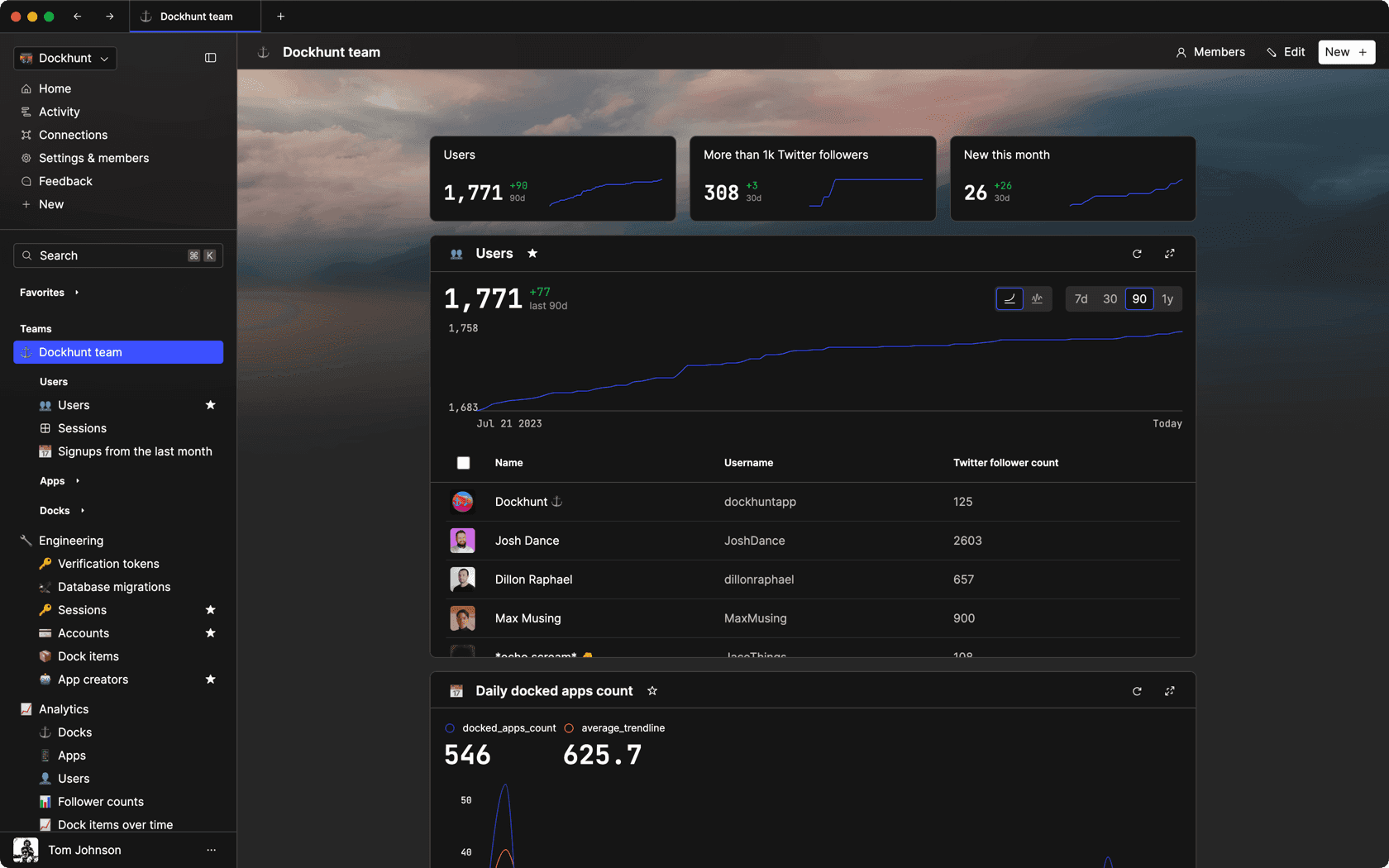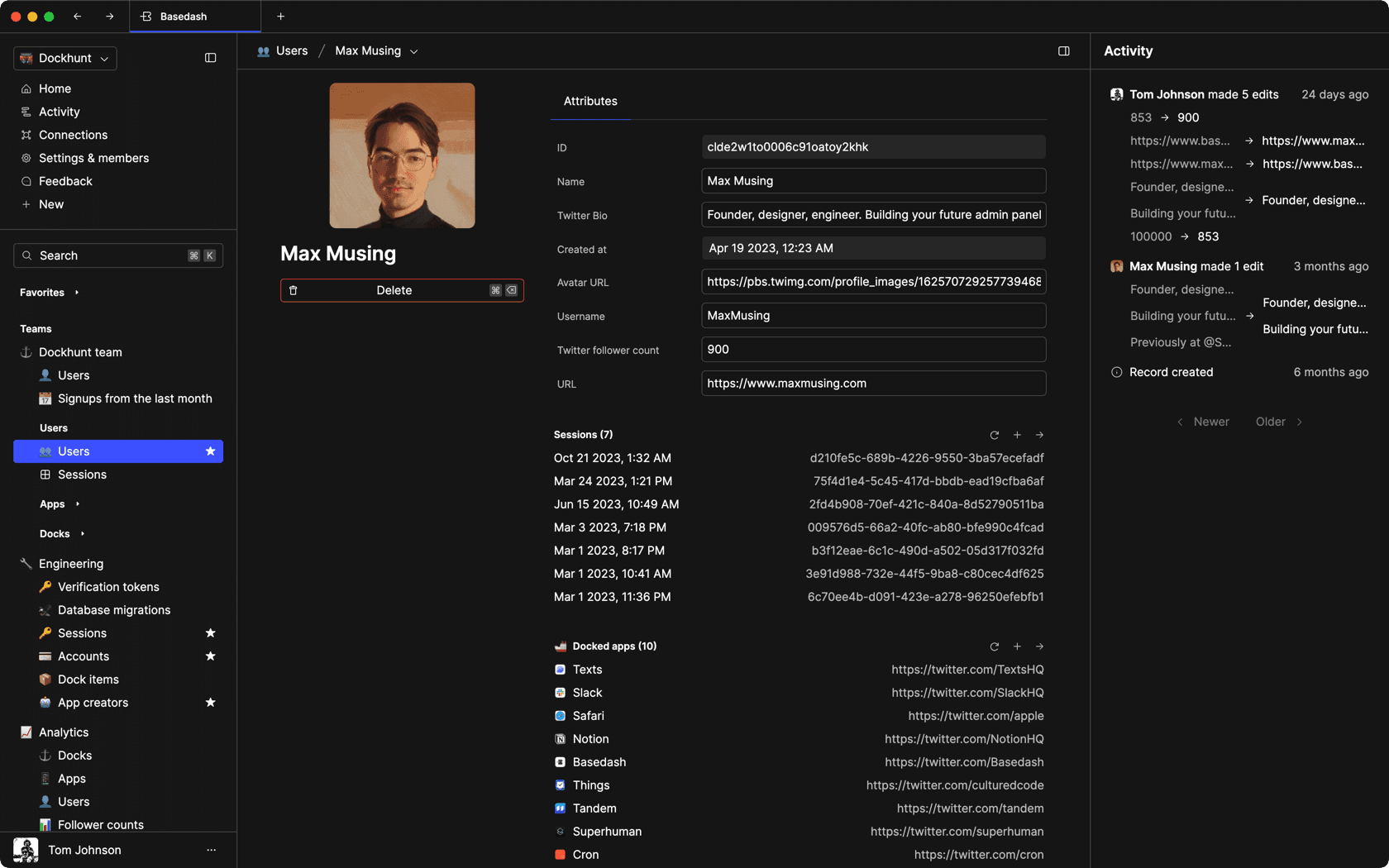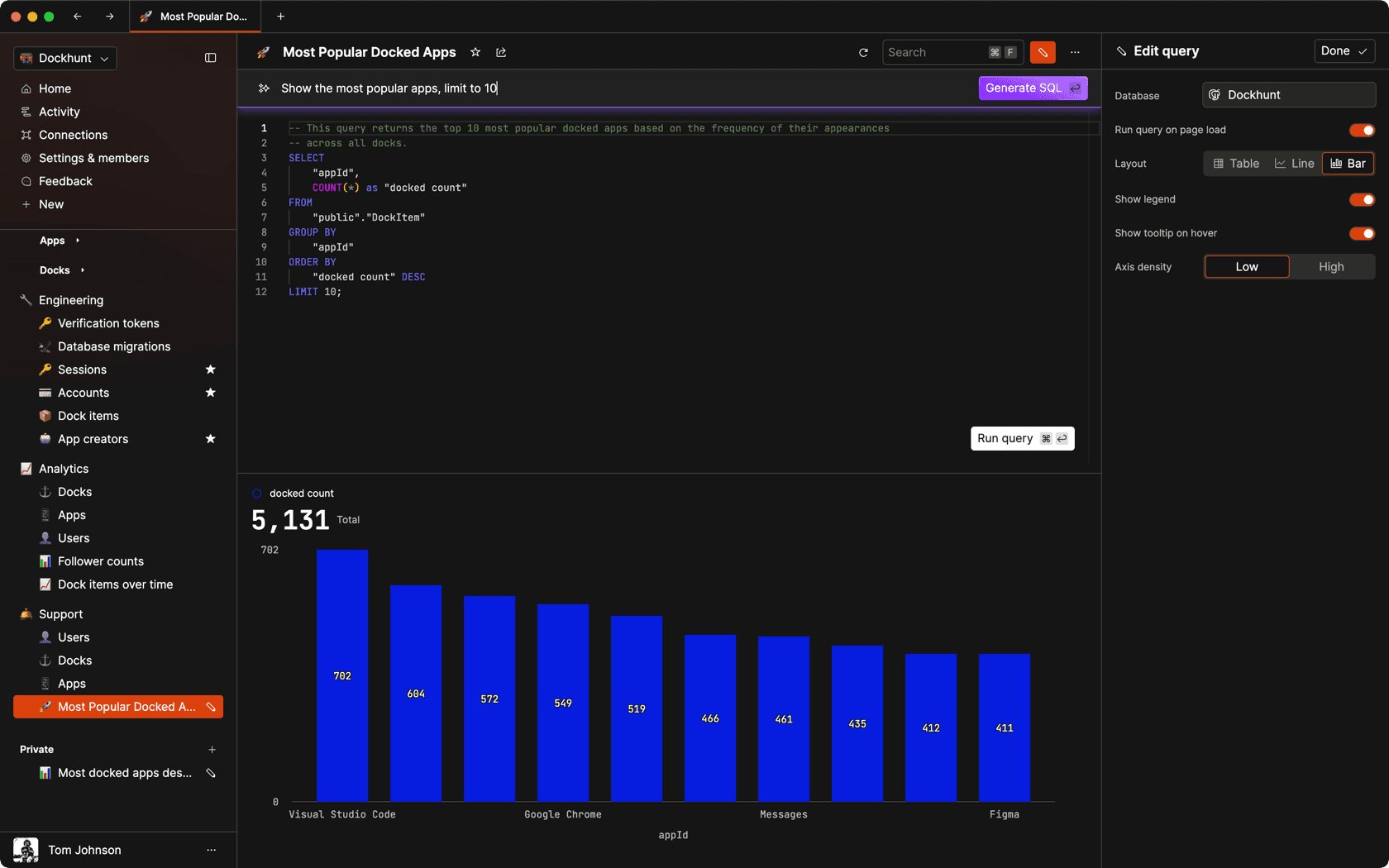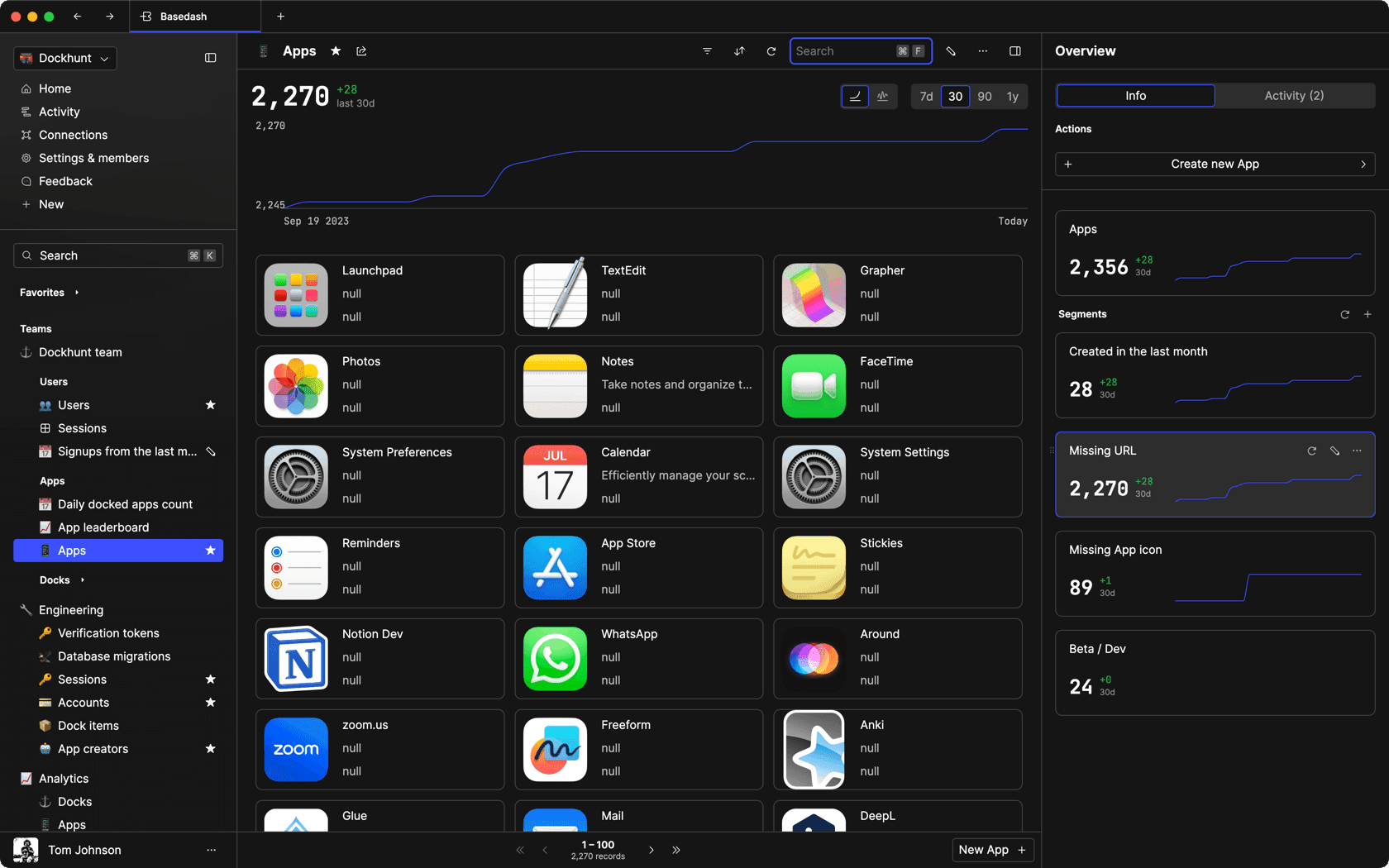### Dashboards and charts

Effortlessly make charts and create a space for your team to work together towards shared goals and metrics.

### SQL composer with AI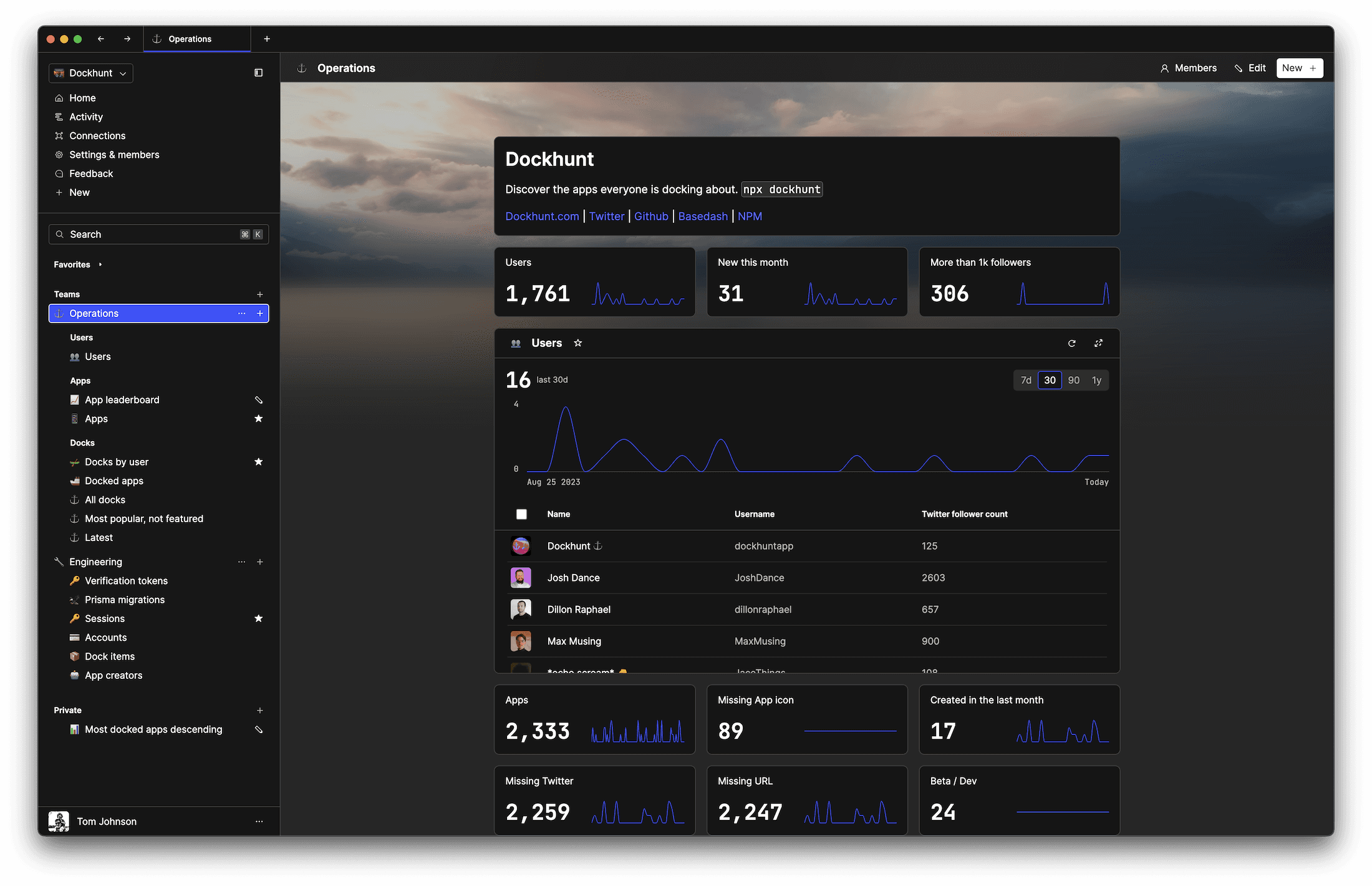SOC II
Type 2 Certified

### Features

Proudly carbon-neutral.SOC II
Type 2 Certified

### Features

Proudly carbon-neutral.SOC II
Type 2 Certified